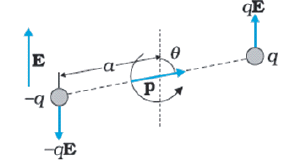An electric dipole is held in a uniform electric field

(i) Show that the net force acting on it is zero

(ii) The dipole is aligned parallel to the field. Find the work done in rotating it through the angle of $180°$.

Concept Videos :-

#8 | Electric Dipoles
#9 | Dipole EFI
#10 | Electric Field Superposition : 2 Electric Dipole
#11 | Electric Dipole(Short) : EFI
#12 | Electric Dipole in Uniform Electric Field : EFI
#13 | Oscillations of a dipole in Uniform Electric Field
#14 | Electric Dipole in Non uniform Electric Field
#15 | Force of interaction between two dipoles

Concept Questions :-

Electric dipole

(i)

$\stackrel{\to }{{\mathrm{F}}_{\mathrm{net}}}=\stackrel{\to }{{\mathrm{F}}_{1}}+\stackrel{\to }{{\mathrm{F}}_{2}}$

$\therefore {\mathrm{F}}_{\mathrm{net}}=0$                                                                                     (1) marks

Alternatively,$\therefore {\mathrm{F}}_{\mathrm{net}}=0$

(ii)

$\mathrm{W}=\mathrm{EP}\left({\mathrm{cos\theta }}_{1}-{\mathrm{cos\theta }}_{2}\right)$                                                                   (1/2) marks

W=2EP                                                                                         (1/2) marks

Difficulty Level:

• 49%
• 23%